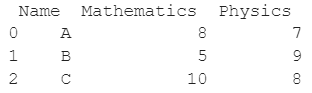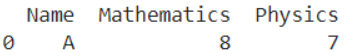# How To Drop A List Of Rows From A Pandas DataFrame?

A DataFrame is the primary data structure of the Pandas library and is commonly used for storing and working with tabular data in Python. A common operation that could be performed on such data is to drop a list of rows from the dataframe in order to remove unwanted information.

To start working with Pandas, we first need to import it by using the below statement:

``import pandas as pd``

## Running Example

Let us understand this operation with the help of an example. Consider the following DataFrame containing 3 students with names A, B, and C and their corresponding marks (out of 10) for two subjects, Mathematics and Physics.Code snippet for generating the above DataFrame:

``````import pandas as pd
# Dictionary for our data
data = {'Name' : ['A', 'B', 'C'], 'Mathematics' : [8, 5, 10], 'Physics' : [7, 9, 8]}
# DataFrame for the dictionary
df = pd.DataFrame(data)
# Printing the DataFrame
print(df)``````

Here, data is a dictionary, we created to initialize the DataFrame. For this, we use the DataFrame() function of the Pandas library which takes the dictionary as an argument and returns the required DataFrame.

Now, let’s say we need to remove the second and third rows i.e the rows with index 1 and 2. The resulting dataframe would look like this:Let us look at different ways of performing this operation on a given DataFrame:

## Delete rows using the drop() function in Pandas dataframe

In this method, we use the dataframe.drop() function to drop rows from a given dataframe based on their index values.

Here, we want to drop the second and third rows. We do this by passing the index values that are obtained by using the index property as a parameter to this function.

By default, the update in the dataframe does not occur in an inplace manner. Let us take a look at the corresponding code snippet and generated output for this method:

``````import pandas as pd
# Dictionary for our data
data = {'Name' : ['A', 'B', 'C'], 'Mathematics' : [8, 5, 10], 'Physics' : [7, 9, 8]}
# DataFrame for the dictionary
df = pd.DataFrame(data)
# Dropping second and third rows
df = df.drop(df.index[[1,2]])
# Printing the new dataframe
print(df)``````

Output :The above operation can also be performed in an in place manner, by setting the inplace parameter of the dataframe.drop() function as True. Let us take a look at the corresponding code snippet and generated output for this method:

``````import pandas as pd
# Dictionary for our data
data = {'Name' : ['A', 'B', 'C'], 'Mathematics' : [8, 5, 10], 'Physics' : [7, 9, 8]}
# DataFrame for the dictionary
df = pd.DataFrame(data)
# Dropping second and third rows
df.drop(df.index[[1,2]], inplace = True)
# Printing the new dataframe
print(df)``````

Output:## Delete rows using the take() function in Pandas dataframe

In this method, we use the take() function to drop rows from a given dataframe based on their index values. Here we want to drop the second and third rows.

Instead of selecting the rows, we want to drop, we can also select rows that we want to keep, here is the first row.

We do this by passing the index values that are obtained by using the dataframe.index property as a parameter to this function. By default, the update in the dataframe does not occur in an inplace manner. Let us take a look at the corresponding code snippet and generated output for this method:

``````import pandas as pd
# Dictionary for our data
data = {'Name' : ['A', 'B', 'C'], 'Mathematics' : [8, 5, 10], 'Physics' : [7, 9, 8]}
# DataFrame for the dictionary
df = pd.DataFrame(data)
# Dropping second and third rows
df = df.take(df.index[])
# Printing the new dataframe
print(df)``````

Output :The above operation can also be performed in an in place manner, by setting the inplace parameter of the dataframe.take() function as True. Let us take a look at the corresponding code snippet and generated output for this method:

``````import pandas as pd
# Dictionary for our data
data = {'Name' : ['A', 'B', 'C'], 'Mathematics' : [8, 5, 10], 'Physics' : [7, 9, 8]}
# DataFrame for the dictionary
df = pd.DataFrame(data)
# Dropping second and third rows
df.take(df.index[], inplace = True)
# Printing the new dataframe
print(df)``````

Output:Delete rows using the isin() function in Pandas dataframe

In this method, we use the isin() function to drop rows from a given dataframe based on their index values.

Here, we want to drop the second and third rows. We do this by applying the .isin() the index values that are obtained by using the index property and the unwanted index values are passed as a parameter here in the form of a list.

Finally, we use the tilde ‘~’ operator to negate this selection. Let us take a look at the corresponding code snippet and generated output for this method:

``````import pandas as pd
# Dictionary for our data
data = {'Name' : ['A', 'B', 'C'], 'Mathematics' : [8, 5, 10], 'Physics' : [7, 9, 8]}
# DataFrame for the dictionary
df = pd.DataFrame(data)
# Dropping second and third rows
df = df[~df.index.isin([1,2])]
# Printing the new dataframe
print(df)``````

Output :## Conclusion

In this topic, we have learned to drop a list of rows from an existing DataFrame, following a running example of test scores of students in different subjects, thus giving us an intuition of how this concept could be applied in real-world situations.

Trending